## Example Questions

### Example Question #3 : Graphing

The graph of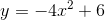passes through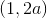in the standard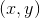coordinate plane. What is the value of?Explanation:

To answer this question, we need to correctly identify where to plug in our given values and solve for.

Points on a graph are written in coordinate pairs. These pairs show thevalue first and thevalue second. So, for this data:means thatis thevalue and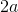is thevalue.

We must now plug in ourandvalues into the original equation and solve. Therefore: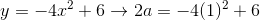We can now begin to solve forby adding up the right side and dividing the entire equation by.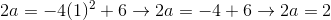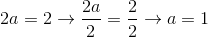Therefore, the value ofis.

### Example Question #1 : How To Graph Complex Numbers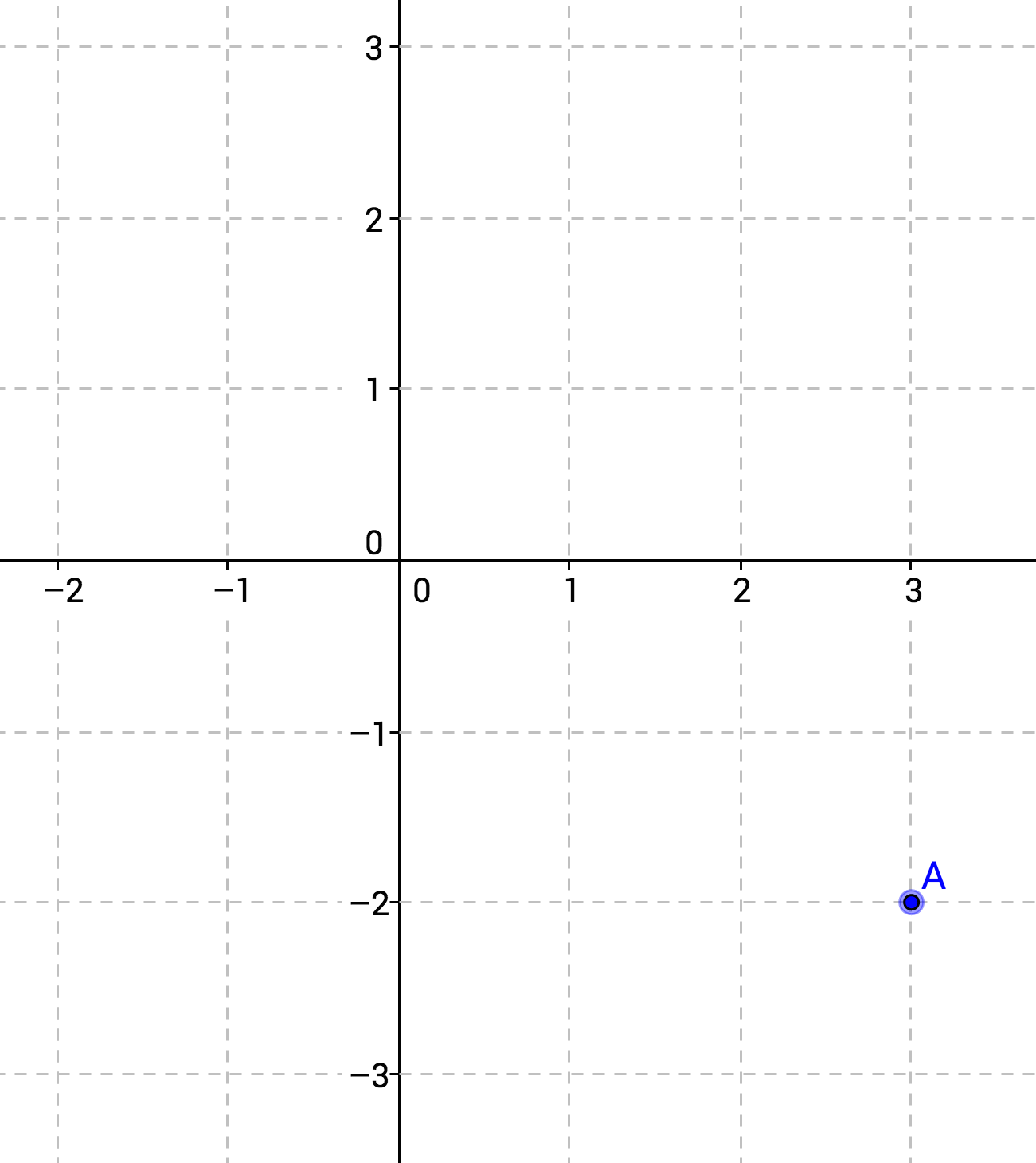Point A represents a complex number.  Its position is given by which of the following expressions?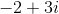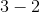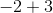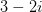Explanation:

Complex numbers can be represented on the coordinate plane by mapping the real part to the x-axis and the imaginary part to the y-axis.  For example, the expression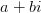can be represented graphically by the point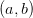.

Here, we are given the graph and asked to write the corresponding expression.not only correctly identifies the x-coordinate with the real part and the y-coordinate with the imaginary part of the complex number, it also includes the necessarycorrectly identifies the x-coordinate with the real part and the y-coordinate with the imaginary part of the complex number, but fails to include the necessary.misidentifies the y-coordinate with the real part and the x-coordinate with the imaginary part of the complex number.misidentifies the y-coordinate with the real part and the x-coordinate with the imaginary part of the complex number.  It also fails to include the necessary.

### Example Question #1 : How To Graph Complex Numbers

Which of the following graphs represents the expression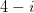?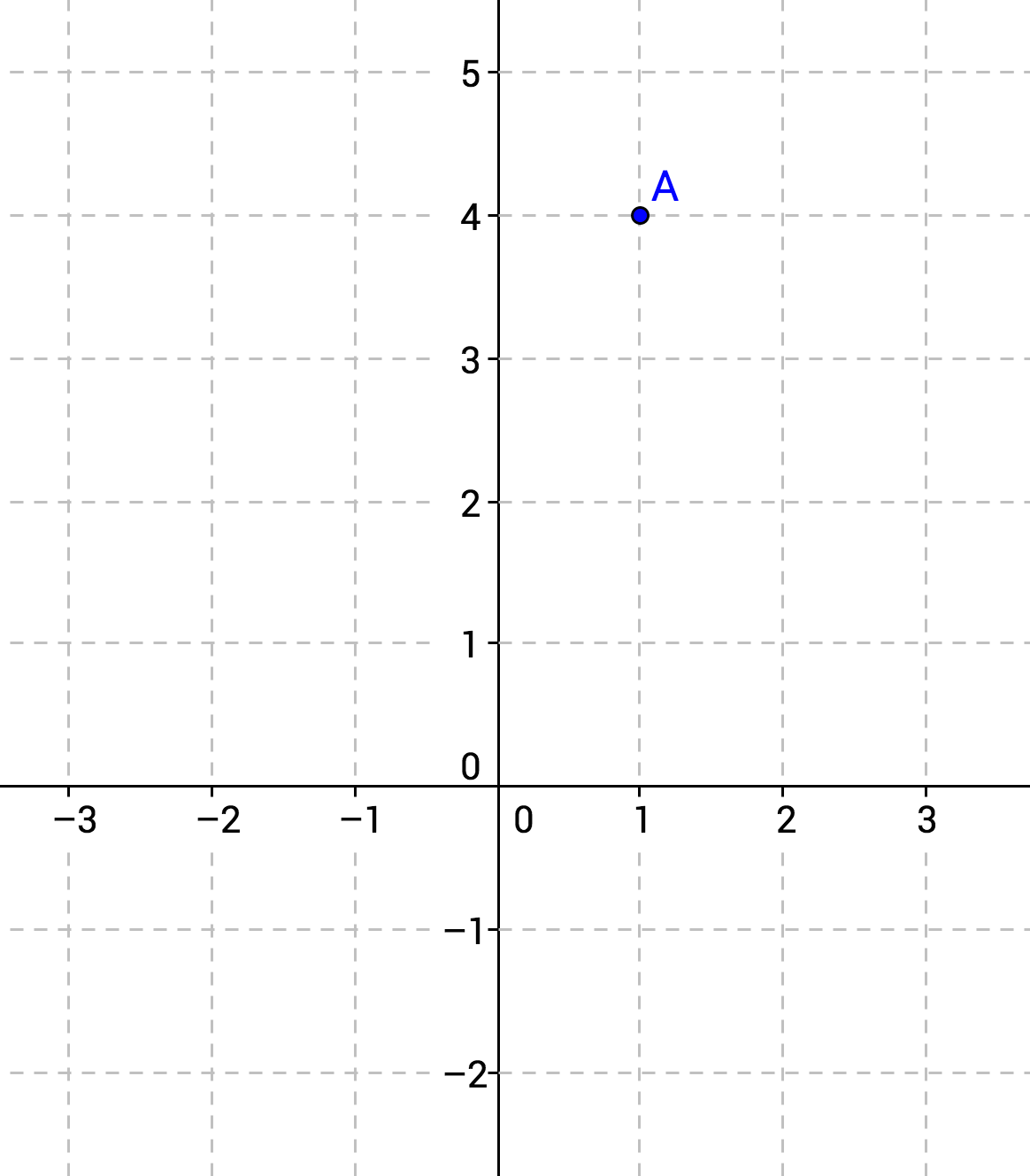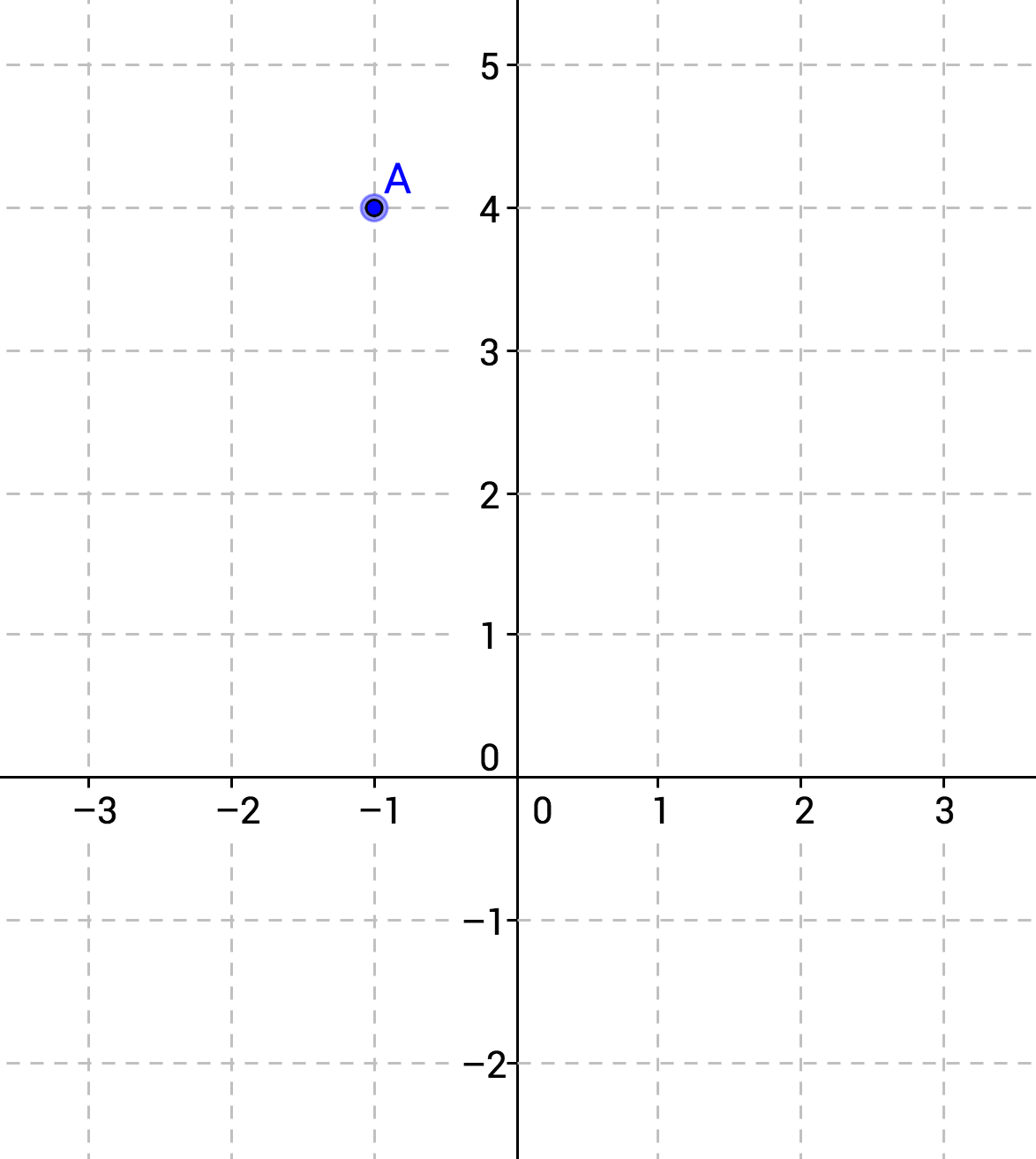Complex numbers cannot be represented on a coordinate plane.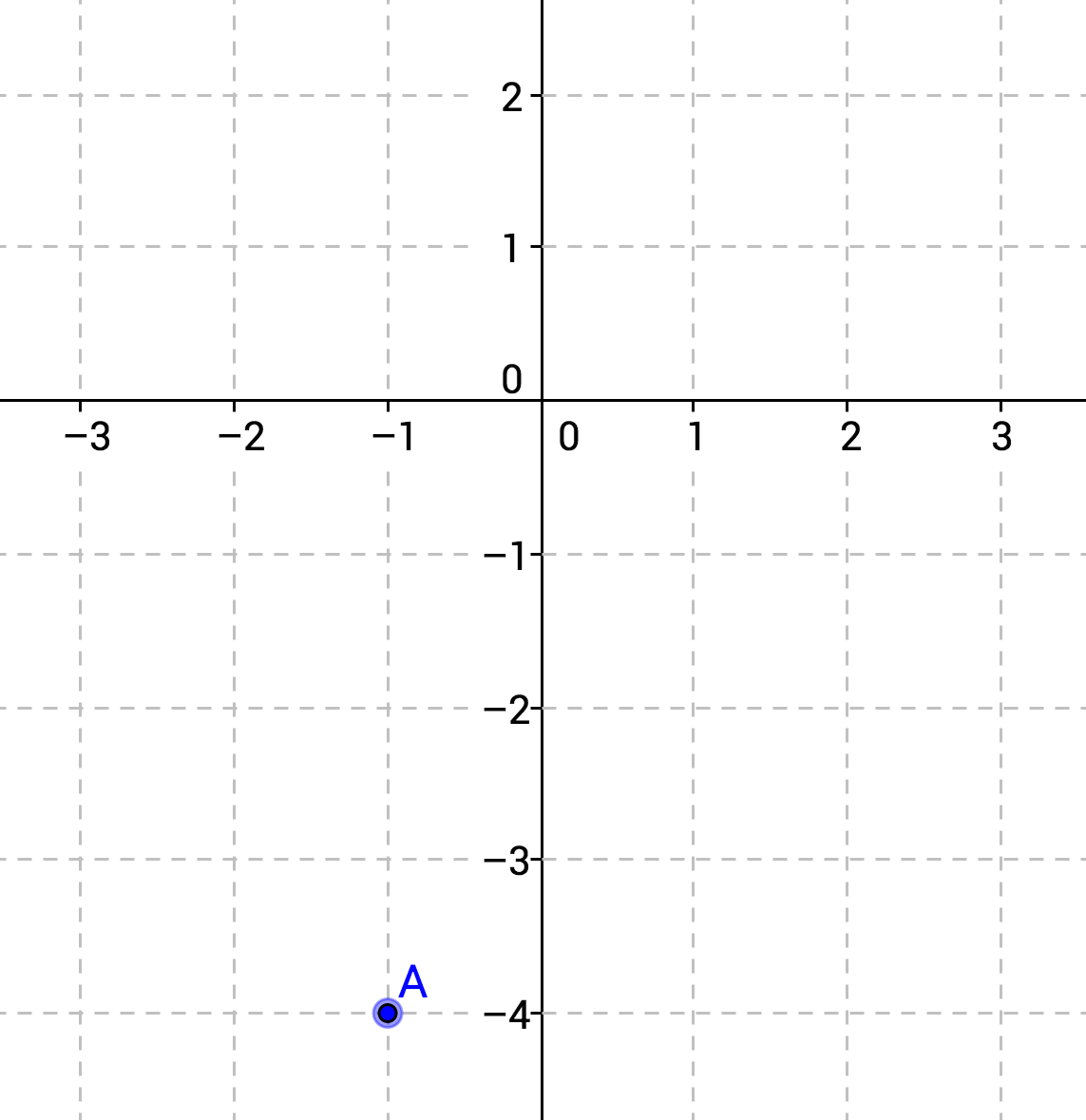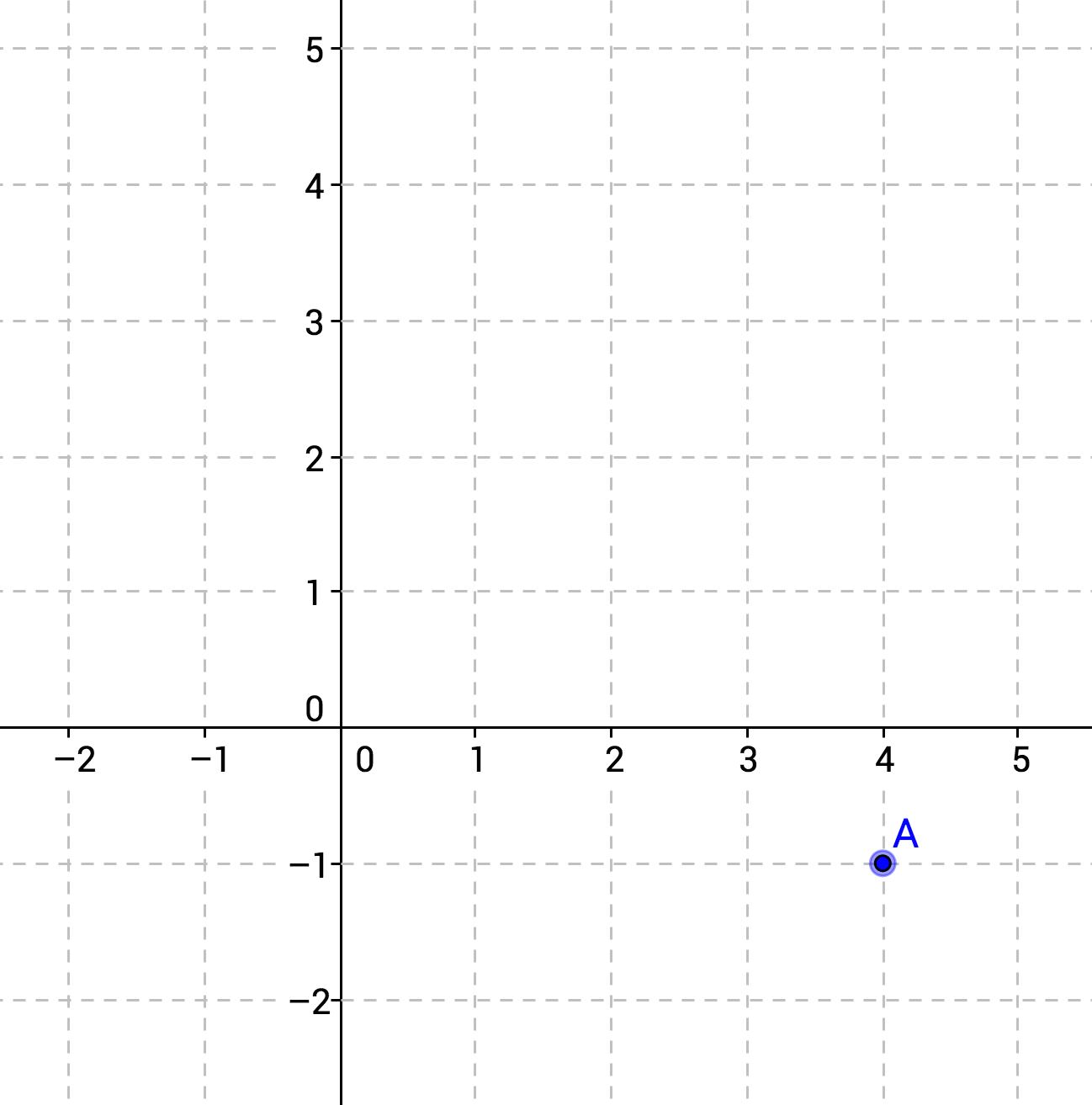Explanation:

Complex numbers can be represented on the coordinate plane by mapping the real part to the x-axis and the imaginary part to the y-axis.  For example, the expressioncan be represented graphically by the point.

Here, we are given the complex numberand asked to graph it.  We will represent the real part,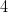, on the x-axis, and the imaginary part,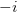, on the y-axis.  Note that the coefficient ofis; this is what we will graph on the y-axis.  The correct coordinates are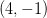.

### Example Question #11 : Graphing

The point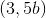is on the graph of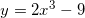. What is the value of?Explanation:

Because points on a graph are written in the form of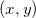, and the point given was, this means thatand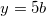.

In order to solve for, these values forandmust be plugged into the given equation. This gives us the following: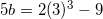We then solve the equation by finding the value of the right side, then dividing the entire equation by 5, as follows: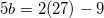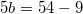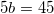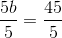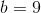Therefore, the value ofis.